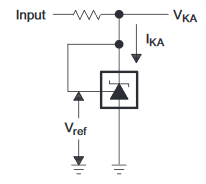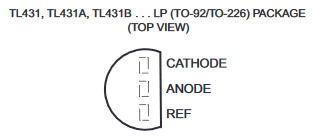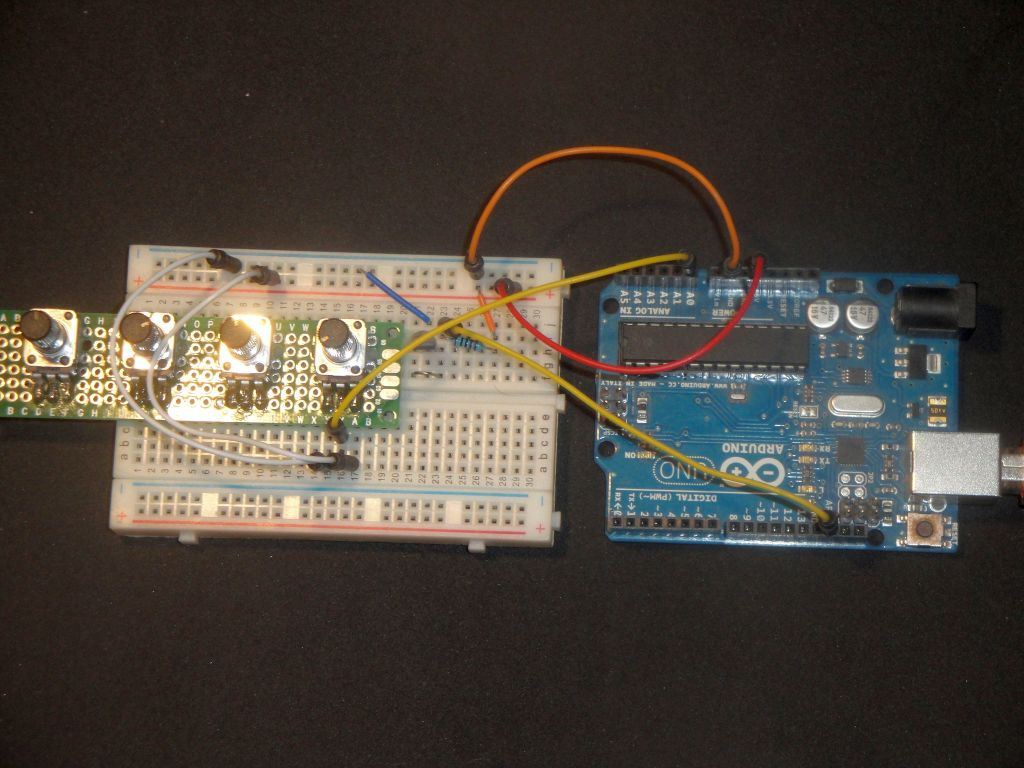# A/D converter - TL431 external voltage reference

Zápisník experimentátora

Hierarchy:

In the articles on the A/D converter we have already addressed the internal voltage reference. The AREF pin enables us to connect external voltage reference that we can adapt to our measurement requirements. The TL431 is a component that provides us with such a reference voltage. This is a cheap piece found in a number of products. For example, in power sources, computers, or LED drivers.In this article, we will use the TL431 in basic connection when providing a 2.5 V reference.## Pins

In the example we will use it on breadboard, so I will describe the package TO-92.

• Cathode - Cathode is connected via a resistor to the input voltage. The resistor limits the current. In the datasheet you will find the recommended values when the component provides a stable reference voltage. Typically, it is recommended to use a resistor of 1.8 k in the 5 V diagrams. If you do not mind a slightly higher current, use resistor 1 k.
• Anode - Anode connects to GND.
• Ref - If we want to use a reference voltage of 2.5 V, we will connect this pin together with the cathode.## Arduino

Arduino and TL431 are connected according to this scheme. The TL431 is connected to the` AREF` pin. Analog measurement is done using a potentiometer on pin `A0`.## Example

In the example I have a potentiometer and TL431 on the breadboard. Through the connecting wires, both components are connected to the Arduino Uno. The potentiometer is connected to pin `A0`. The TL431 is connected to the pin `AREF`.Use the function analogReference to set the external reference voltage. An analog value is then measured on the potentiometer and sent to the serial port.

``````const double tl431_ref = 2.5;
int value;
double value_v;

void setup() {
analogReference(EXTERNAL);

Serial.begin(115200);
}

void loop() {
Serial.print("value = ");
Serial.print(value);
value_v = value * tl431_ref / 1023;
Serial.print(", ");
Serial.print(value_v);
Serial.println(" V");
delay(1000);
}``````

## Source code

The source code is located on the GitHub server.

16.11.2018